Bachelor of computertational mathematics## 项目背景

`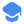专业排名451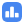大学纽芬兰纪念大学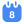开学时间1,5,9月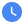课程学制4年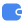学费11460.00/CAD## 专业介绍## 推荐顾问## 世界排名

2020年

451

2018年

351## 语言要求

PTE
Listen
--
--
--
Speak
--
--
--
--
--
--
Write
--
--
--

6.5
79
--## 学术要求## 课程设置

1.必修课:
Math 1000 – Calculus I微积分I
Math 1001 – Calculus II微积分II
Math 1052 – Mathematics for Business商业数学
Math 1090 – Algebra and Trigonometry代数和三角学
Math 2000 – Calculus III微积分III
Math 2050 – Linear Algebra I线性代数I
Math 2051 – Linear Algebra II *线性代数II
Math 3240 – Applied Graph Theory应用图论
Math 3340 – Introductory Combinatorics *组合初学者
Math 3370 – Introductory Number Theory *入门数论
Math 4242 – Algorithms and Complexity算法和复杂性
Computer Science 1510 - An Introduction to Programming for Scientific Computing计算机科学1510-科学计算编程简介
Statistics 2550 – Statistics for Science Students理科学生的统计
Math 1053 – Classical Mathematics *古典数学
MATH 2130 Technical Writing in Mathematics数学技术写作
MATH 2260 Ordinary Differential Equations 常微分方程
MATH 2320 Discrete Mathematics 离散数学
MATH 2330 Euclidean Geometry 欧几里得几何
MATH 3000 Real Analysis I真实分析I
MATH 3132 Numerical Analysis I数值分析I
MATH 3240 Applied Graph Theory应用图论
MATH 3340 Introductory Combinatorics入门组合
MATH 3370 Introductory Number Theory介绍性数论
MATH 4340 Combinatorial Analysis 组合分析Master of Mathematics (MMath) in Computer ScienceThe Master of Financial MathematicsBachelor of computertational mathematicsMSc in Mathematics (Course-Based Option)